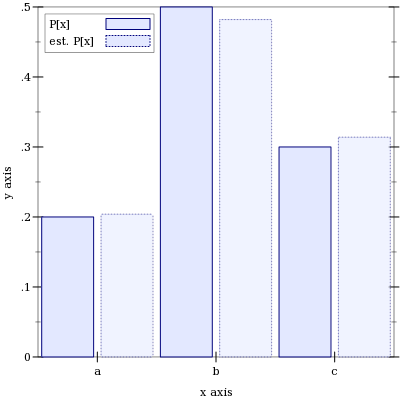#### 9.3Finite Distribution Families

##### 9.3.1Unordered Discrete Distributions

 syntax
 procedure(discrete-dist xs) → (Discrete-Dist A) xs : (Sequenceof A) (discrete-dist xs ws) → (Discrete-Dist A) xs : (Sequenceof A) ws : (Sequenceof Real)
 procedure(discrete-dist-values d) → (Listof A) d : (Discrete-Dist A)
 procedure d : (Discrete-Dist A)
Represents families of unordered, discrete distributions over values of type A, with equality decided by equal?.

The weights in ws must be nonnegative, and are treated as unnormalized probabilities. When ws is not given, the values in xs are assigned uniform probabilities.

The type (Discrete-Dist A) is a subtype of (dist A A). This means that discrete distribution objects are unordered, and thus have only a pdf and a procedure to generate random samples.

Examples:

> (define xs '(a b c))
> (define d (discrete-dist xs '(2 5 3)))
> (define n 500)
> (define h (samples->hash (sample d n)))
 > (plot (list (discrete-histogram (map vector xs (map (distribution-pdf d) xs)) #:x-min 0 #:skip 2 #:label "P[x]") (discrete-histogram (map vector xs (map (λ (x) (/ (hash-ref h x) n)) xs)) #:x-min 1 #:skip 2 #:line-style 'dot #:alpha 0.5 #:label "est. P[x]")))